###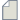Author Topic: Poisson Ratio nanotube  (Read 516 times)

0 Members and 1 Guest are viewing this topic.

####sadegh

• New ATK user
•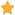• Posts: 6
• Country: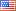• Reputation: 0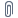##### Poisson Ratio nanotube
« on: December 28, 2020, 17:00 »
Hi.

I am trying to extract the plot such as stress vs strain, radius change vs strain, and energy vs strain for a nanotube that is strained in z (length) direction. I combined the python codes found in the forum and the code in the tutorial which is attached. As you see the nanotube is under 1.0 (no strain), 1.01 (1 percent strain), and 1.02 (2 percent strain). I was wondering how I can save/print the total energy of each strained structure after optimization and plot it against strain?
Furthermore, I would like to save/print the radius change (final radius-initial/initial) of the 3 strained structures versus strain in order to find the poison ratio.
One last question, what is the correct command for finding the distance between two atoms (for example carbon #5 and carbon #10).

####Petr Khomyakov

• QuantumATK Staff
• Supreme ATK Wizard
•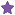• Posts: 1286
• Country:• Reputation: 24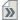##### Re: Poisson Ratio nanotube
« Reply #1 on: January 8, 2021, 11:37 »
You can definitely calculate and print out total energy as given in the usage examples in the QuantumATK manual, https://docs.quantumatk.com/manual/Types/TotalEnergy/TotalEnergy.html. You can print other quantities (strain value, radius and so on) in a similar manner. Plotting can be done, e.g., using pyplot, https://matplotlib.org/3.1.1/api/_as_gen/matplotlib.pyplot.html, https://matplotlib.org/3.2.1/api/_as_gen/matplotlib.pyplot.plot.html, that requires some python scripting and then one can execute the script by sending it to the Job Manager,

####Anders Blom

• QuantumATK Staff
• Supreme ATK Wizard
•• Posts: 5012
• Country:• Reputation: 85##### Re: Poisson Ratio nanotube
« Reply #2 on: January 13, 2021, 20:07 »
As for distance between two atoms, you write Carbon #5 and #10, but I am not sure if you mean atoms #5 and #10 (which happen to be Carbon) or the 5th and 10th Carbon atoms? Anyway:
Code
``````# Coordinates of all atoms
coords = configuration.cartesianCoordinates()
# Distance between atom 5 and 10 (note that we start counting from 0!)
numpy.linalg.norm(coords-coords)
``````
If you need to search for the indices of atoms, you could try (to find the 5th carbon atom):
Code
``````all_carbon = numpy.where(numpy.array(configuration.elements())==Carbon)
c5 = all_carbon    # again, starting at 0 so  is 5th carbon atom!
``````
If you have other needs to identify certain atoms, I would recommend using tags to label them. So let's say you have tagged two atoms, atom5 and atom10 using the Builder (or a script), then you can find the distance between them using
Code
``````c5 = configuration.indicesFromTags("atom5")
c10 = configuration.indicesFromTags("atom10")
distance = numpy.linalg.norm(coords[c5]-coords[c10])
``````